# A Riemann Solver For the Isothermal Equations

## by John Weatherwax

Introduction
Here you'll find code in FORTRAN implementing a Riemann solver for the Isothermal equations of gas dynamics. The Isothermal equations arise as a simplification to the full Euler equations of gas dynamics when one assumes that all motion occurs at constant temperature. The resulting equations are given by the following system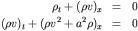The system above is closed in terms of the two conservative variables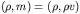or the two primitive variables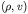A Riemann problem is the solution to the above partial differential equations with a discontinuous initial condition given by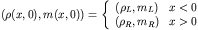The Riemann solver itself is contained in the file "riemann.f", but a simple driver application is compiled when one builds the package. The driver application is called "ne.out" and reads its input from a text file called newt.inp. In this text file one specifies the left and right states, a Courant like number for use in printing, and some flags that control output. In the example below the code solves a Riemann problem with initial conditions given by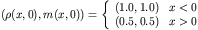The current flags settable in newt.inp are (they can be turned on or off)
• PRINT_NEWTON: Prints to the screen the initial state and the density updates computed as the newton iterations converge (at each timestep) as the intersection of the two wave curves is found in the density momentum plane. Note: the intersection of the wave curves is found by considering density to be an independend variable and momentum the dependent variable.
• PRINT_NEWTON_CONV: Prints the convergence steps of the newton iterations into two files "rm_conv_l_test.dat", and "rm_conv_r_test.dat" representing the newton iterates on each of the two wave curves (left and right) in the density momentum space respectively.
• PRINT_RP: Prints a summary of all the wave produced for the specific Riemann problem to be solved. This is saved in the file "RP_test.dat". The information includes the states between the two waves, the wave speeds, the wave types (shock, or rarefaction fan) and a flag variable denoting the "significance" of the waves a boolean variable denoting a waves strengh (strong or weak).
• PRINT_WC: Prints the left and right wave curves in the (density,momentum) plane and the left and right Riemann invariants (in the file iLiR_curv_{l,r}_test.dat). The ordering of the variables in the files is given by the ordering in the filename. We define the left and right Riemann invarients for the isothermal equations below.
• PRINT_INIT_WC: Currently not used.
As an output from this Riemann solver we additionally produce the left and right Riemann invarients for this system. The left going Riemann invariant is constant on paths governed by the left facing characteristics (ones traveling on dx/dt=u-a) and is also called 2-Riemann invariant since it is constant across 2-integral waves. For the Isothermal equations they are defined by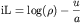The right Riemann invariant is constant on paths governed by the right going characteristics (dx/dt=u+a) and is also called a 1-Riemann invariant since it is constant across 1-integral waves. For the Isothermal equations they are defined by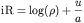Note that these definitions of the Riemann invariants are a constant multiple of 1/a of those found in the book:
Finite Volume Methods For Hyperbolic Problems
by Randall J. LeVeque
As an example consider the Riemann problem with initial conditions given by Solving the above Riemann problem produces the following left and right wave curves (the wave curve through the left state is the colored blue and the wave curve through the right state is colored red)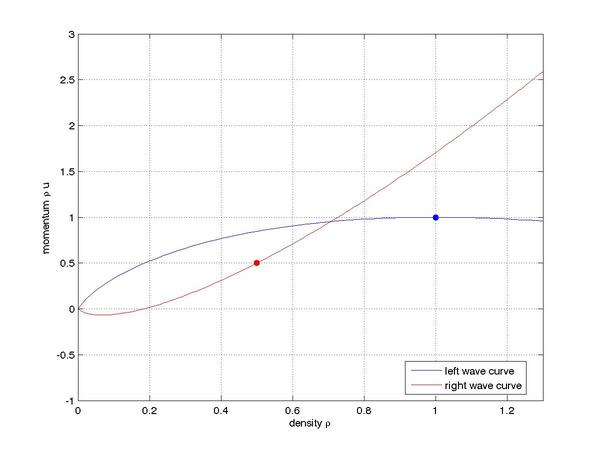We next plot the waves produced in density at one unit of time from the initial conditions giving: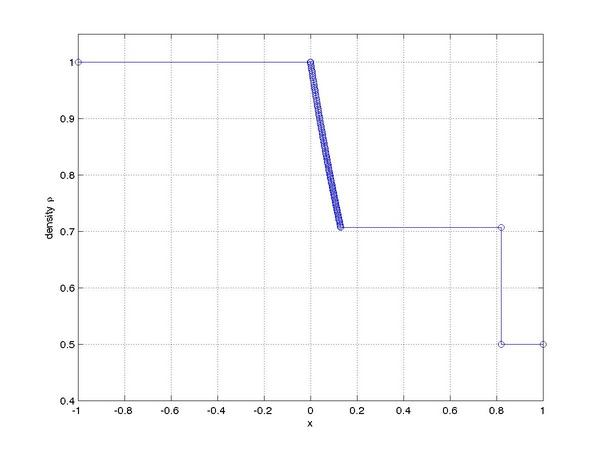The corresponding plot of momentum at one unit of time after the initial conditions looks like: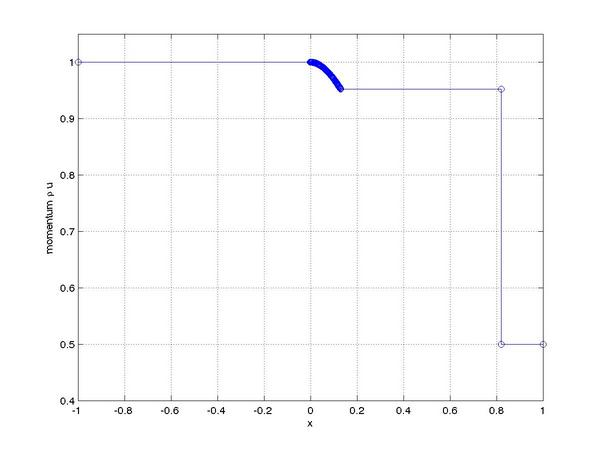When the delivered code is built, it contains an additional program to compute the wave curves centered at a given state. The program is called "twc.out" Finally, here is the code
As always, I am interested in hearing back if any errors are found to exist.
John Weatherwax